# Chapter 7: Bayesian modeling for point referenced spatio-temporal data

University of Southampton

### 2022-03-30

Abstract

This file contains all the code for reproducing the figures in Chapter 7.

``````## Note the start time
start.time<-proc.time()
figurepath <- "figures/chap7figures/"
filepath <- "txttables/"
mappath <- "mapfiles/"
longrun <- FALSE
f2 <- y8hrmax~xmaxtemp+xwdsp+xrh
colpalette <- c("dodgerblue4",  "dodgerblue2", "firebrick2",   "firebrick4",   "purple")
longrun <- FALSE
``````library(bmstdr)
## Warning in .recacheSubclasses(def@className, def, env): undefined subclass
## "numericVector" of class "Mnumeric"; definition not updated
library(ggplot2)
library(akima)
library(tidyr)
library(RColorBrewer)
library(ggpubr)
library(spdep)
library(GGally)
library(geoR)
library(fields)
library(doBy)
library(xtable)``````

# 1 Code to reproduce Table 7.1

``````
##   s.index Longitude Latitude     utmx    utmy Year Month Day y8hrmax xmaxtemp
## 1       1   -73.757   42.681 601838.1 4726140 2006     7   1   53.88 27.85772
## 2       1   -73.757   42.681 601838.1 4726140 2006     7   2   57.13 30.11563
## 3       1   -73.757   42.681 601838.1 4726140 2006     7   3   72.00 30.00001
## 4       1   -73.757   42.681 601838.1 4726140 2006     7   4   36.63 27.89656
## 5       1   -73.757   42.681 601838.1 4726140 2006     7   5   42.63 25.65698
## 6       1   -73.757   42.681 601838.1 4726140 2006     7   6   30.88 24.61968
##      xwdsp      xrh
## 1 5.459953 2.766221
## 2 8.211767 3.197750
## 3 4.459581 3.225186
## 4 3.692225 4.362334
## 5 4.374314 3.950320
## 6 4.178086 3.420533

M1 <- Bsptime(model="lm", formula=f2, data=nysptime, scale.transform = "SQRT",
N=5000)
## ##
## # Total time taken:: 0.01  - Sec.
summary(M1)
##
##  The lm model has been fitted using bmstdr code in R.
## Call:
## Bsptime(formula = f2, data = nysptime, model = "lm", scale.transform = "SQRT",
##     N = 5000)
## ##
## # Total time taken:: 0.01  - Sec.
## Model formula
## y8hrmax ~ xmaxtemp + xwdsp + xrh
##
##
## Parameter Estimates:
##               mean    sd   2.5%  97.5%
## (Intercept)  2.223 0.240  1.754  2.693
## xmaxtemp     0.175 0.007  0.162  0.188
## xwdsp        0.089 0.012  0.065  0.113
## xrh         -0.173 0.028 -0.228 -0.119
## sigma2       0.555 0.019  0.519  0.593
M1\$tn
##  0
M1\$sn
##  1736
##
plot(M1)
##
##  No other plots implemented for this model fitting method.
##
##  Note that the residuals are provided on the transformed scale. Please see the scale.transform argument.
##
## Summary of the residuals
##      Min.   1st Qu.    Median      Mean   3rd Qu.      Max.      NA's
## -2.364104 -0.527171  0.001326 -0.002186  0.501164  2.514540        24
## tn has not been supplied in residuals and it is
##     not possible to figure this out. Hence a time series plot of the residuals
##     has not been provided here.
##
##  Note that the residuals are provided on the transformed scale.
##     Please see the scale.transform argument.``````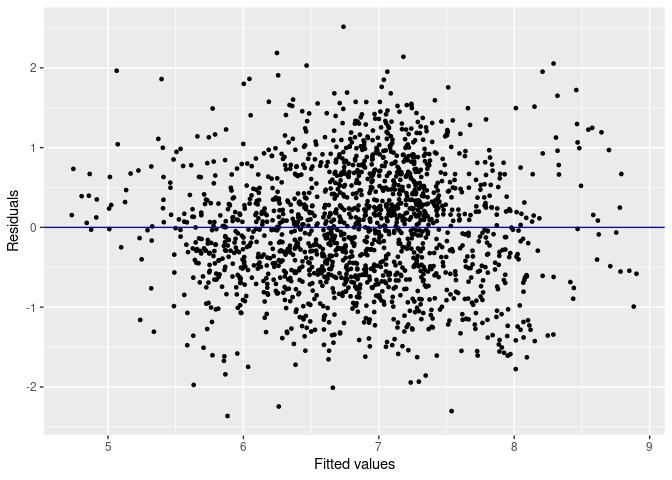``````a <- residuals(M1, numbers=list(sn=28, tn=62))
##
##  Note that the residuals are provided on the transformed scale. Please see the scale.transform argument.
##
## Summary of the residuals
##      Min.   1st Qu.    Median      Mean   3rd Qu.      Max.      NA's
## -2.364104 -0.527171  0.001326 -0.002186  0.501164  2.514540        24``````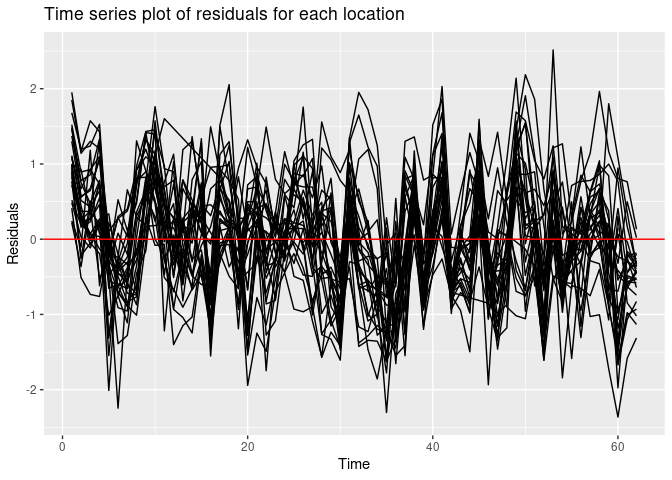``````a <- residuals(M1, numbers=list(sn=28))
##
##  Note that the residuals are provided on the transformed scale. Please see the scale.transform argument.
##
##
## Summary of the residuals
##      Min.   1st Qu.    Median      Mean   3rd Qu.      Max.      NA's
## -2.364104 -0.527171  0.001326 -0.002186  0.501164  2.514540        24
## tn has not been supplied in residuals and it is
##     not possible to figure this out. Hence a time series plot of the residuals
##     has not been provided here.
## Spatio-temporal model fitting

M2 <- Bsptime(model="separable", formula=f2, data=nysptime, scale.transform = "SQRT",
coordtype="utm", coords=4:5,  N=5000)
## ##
## # Total time taken:: 0.01  - Sec.
names(M2)
##   "params"           "fit"              "max.d"            "fitteds"
##   "phi.s"            "phi.t"            "residuals"        "sn"
##   "tn"               "formula"          "scale.transform"  "package"
##  "model"            "call"             "computation.time"
summary(M2)
##
##  The separable model has been fitted using bmstdr code in R.
## Call:
## Bsptime(formula = f2, data = nysptime, model = "separable", coordtype = "utm",
##     coords = 4:5, scale.transform = "SQRT", N = 5000)
## ##
## # Total time taken:: 0.01  - Sec.
## Model formula
## y8hrmax ~ xmaxtemp + xwdsp + xrh
##
##
## Parameter Estimates:
##               mean    sd   2.5%  97.5%
## (Intercept)  0.191 1.740 -3.219  3.602
## xmaxtemp     0.259 0.037  0.187  0.331
## xwdsp        0.010 0.035 -0.059  0.079
## xrh         -0.011 0.109 -0.226  0.203
## sigma2      12.189 0.414 11.405 13.027
M2\$phi.s
##  0.005072598
M2\$phi.t
##  0.0483871
table7.1 <- cbind(M1\$params, M2\$params)
dput(table7.1, file=paste0(filepath, "table7.1.txt"))
print(round(table7.1, 2))
##              mean   sd  2.5% 97.5%  mean   sd  2.5% 97.5%
## (Intercept)  2.22 0.24  1.75  2.69  0.19 1.74 -3.22  3.60
## xmaxtemp     0.17 0.01  0.16  0.19  0.26 0.04  0.19  0.33
## xwdsp        0.09 0.01  0.06  0.11  0.01 0.04 -0.06  0.08
## xrh         -0.17 0.03 -0.23 -0.12 -0.01 0.11 -0.23  0.20
## sigma2       0.55 0.02  0.52  0.59 12.19 0.41 11.40 13.03
# xtable(table7.1, digits=2)
# a <- phichoicep()``````
``````valids <-  c(8,11,12,14,18,21,24,28)
vrows <-  which(nysptime\$s.index%in% valids)

M2.1 <- Bsptime(model="separable",  formula=f2, data=nysptime,
validrows=vrows, coordtype="utm", coords=4:5,
phi.s=0.005, phi.t=0.05, scale.transform = "SQRT")
## ##
## # Total time taken:: 7.61  - Sec.``````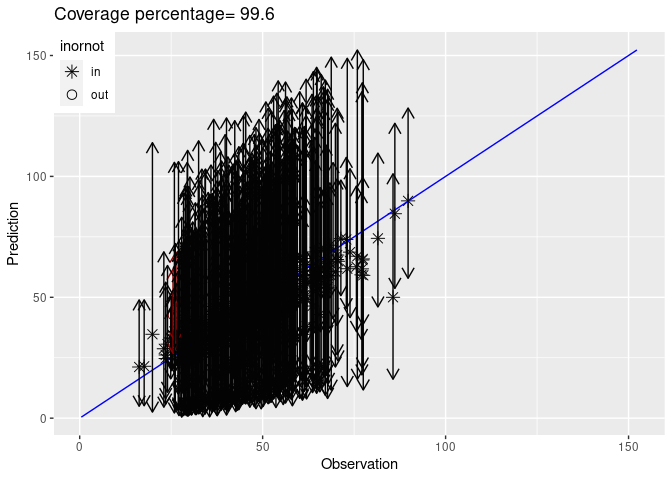``````names(M2.1)
##   "params"           "fit"              "max.d"            "fitteds"
##   "stats"            "yobs_preds"       "valpreds"         "phi.s"
##   "phi.t"            "residuals"        "sn"               "tn"
##  "formula"          "scale.transform"  "package"          "model"
##  "call"             "computation.time"
dim(M2.1\$valpreds)
##  2000  496
u <- get_validation_summaries(M2.1\$valpreds)
dim(u)
##  496   5
##   meanpred    sdpred medianpred      low       up
## 1 67.86876 11.203631   66.89214 48.59173 91.42743
## 2 66.02045 11.012413   65.57377 45.88358 89.08762
## 3 58.56413 10.636774   58.43256 39.25781 80.54063
## 4 58.23229 10.559617   57.67607 39.85306 80.69852
## 5 42.09230  8.741677   41.86654 26.63715 59.88194
## 6 31.87050  7.653939   31.41477 18.79720 48.34924
M1 <- Bsptime(model="lm", formula=f2, data=nysptime,
validrows=vrows, scale.transform = "SQRT")
## ##
## # Total time taken:: 7.14  - Sec.``````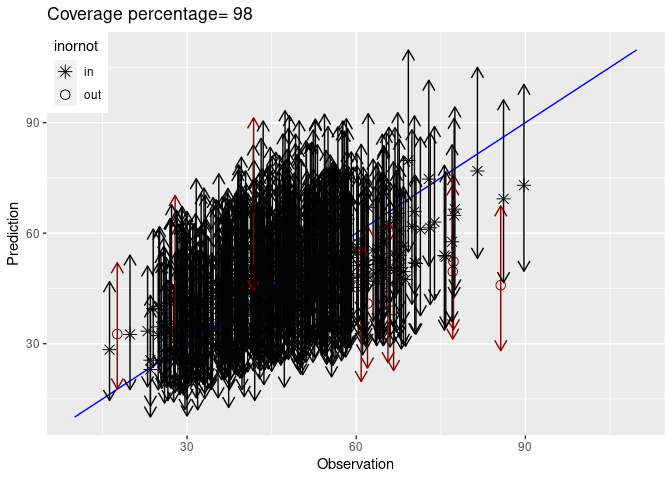``````summary(M1)
##
##  The lm model has been fitted using bmstdr code in R.
## Call:
## Bsptime(formula = f2, data = nysptime, model = "lm", validrows = vrows,
##     scale.transform = "SQRT")
## ##
## # Total time taken:: 7.14  - Sec.
## Model formula
## y8hrmax ~ xmaxtemp + xwdsp + xrh
##
##
## Parameter Estimates:
##               mean    sd   2.5%  97.5%
## (Intercept)  2.303 0.293  1.728  2.878
## xmaxtemp     0.174 0.008  0.159  0.190
## xwdsp        0.089 0.015  0.061  0.118
## xrh         -0.190 0.034 -0.256 -0.125
## sigma2       0.588 0.024  0.543  0.636
##
## Validation Statistics:
##   rmse    mae   crps    cvg
##  9.367  7.549  5.627 97.951
summary(M2.1)
##
##  The separable model has been fitted using bmstdr code in R.
## Call:
## Bsptime(formula = f2, data = nysptime, model = "separable", coordtype = "utm",
##     coords = 4:5, validrows = vrows, scale.transform = "SQRT",
##     phi.s = 0.005, phi.t = 0.05)
## ##
## # Total time taken:: 7.61  - Sec.
## Model formula
## y8hrmax ~ xmaxtemp + xwdsp + xrh
##
##
## Parameter Estimates:
##               mean    sd   2.5%  97.5%
## (Intercept)  0.164 1.652 -3.074  3.402
## xmaxtemp     0.258 0.036  0.188  0.328
## xwdsp        0.002 0.034 -0.065  0.068
## xrh          0.008 0.107 -0.201  0.217
## sigma2      10.334 0.415  9.552 11.179
##
## Validation Statistics:
##   rmse    mae   crps    cvg
##  6.497  5.013 10.670 99.590
plot(M2.1)
##
##  No other plots implemented for this model fitting method.
##
##  Note that the residuals are provided on the transformed scale. Please see the scale.transform argument.
## Validation has been performed. The residuals include the validation observations as well.
##     Expect the return value to be of the same length as the supplied data frame.
##
## Summary of the residuals
##     Min.  1st Qu.   Median     Mean  3rd Qu.     Max.     NA's
## -3.11069 -0.97055 -0.47227 -0.47438  0.04332  2.13322       24
##
##  Note that the residuals are provided on the transformed scale.
##     Please see the scale.transform argument.
## Validation has been performed
## The fitted values include the validation observations as well.
## Expect the return value to be of the same length as the supplied data frame.``````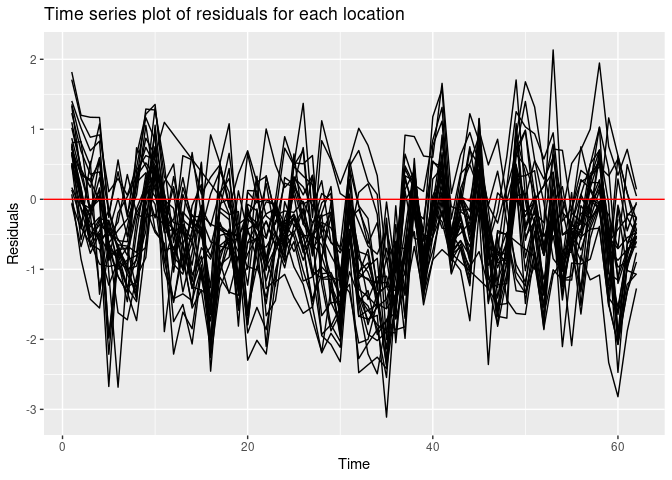``````##
## Validation Statistics:``````